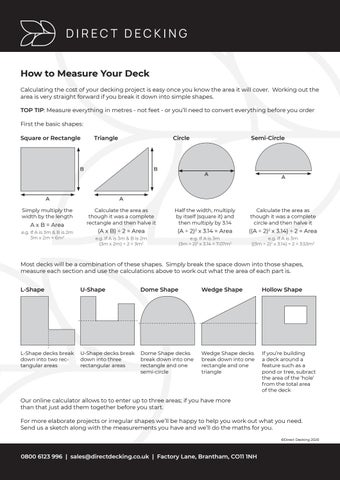__MAIN_TEXT__How to Measure Your Deck Calculating the cost of your decking project is easy once you know the area it will cover. Working out the area is very straight forward if you break it down into simple shapes. TOP TIP: Measure everything in metres - not feet - or you’ll need to convert everything before you order First the basic shapes: Square or Rectangle

Triangle

Circle

B

B

Semi-Circle

A

A

A

A

Simply multiply the width by the length

Calculate the area as though it was a complete rectangle and then halve it

Half the width, multiply by itself (square it) and then multiply by 3.14

Calculate the area as though it was a complete circle and then halve it

(A x B) ÷ 2 = Area

(A ÷ 2)2 x 3.14 = Area

((A ÷ 2)2 x 3.14) ÷ 2 = Area

e.g. If A is 3m & B is 2m (3m x 2m) ÷ 2 = 3m2

e.g. If A is 3m (3m ÷ 2)2 x 3.14 = 7.07m2

e.g. If A is 3m ((3m ÷ 2)2 x 3.14) ÷ 2 = 3.53m2

A x B = Area e.g. If A is 3m & B is 2m 3m x 2m = 6m2

Most decks will be a combination of these shapes. Simply break the space down into those shapes, measure each section and use the calculations above to work out what the area of each part is. L-Shape

U-Shape

Dome Shape

Wedge Shape

Hollow Shape

L-Shape decks break down into two rectangular areas

U-Shape decks break down into three rectangular areas

Dome Shape decks break down into one rectangle and one semi-circle

Wedge Shape decks break down into one rectangle and one triangle

If you’re building a deck around a feature such as a pond or tree, subract the area of the ‘hole’ from the total area of the deck

Our online calculator allows to to enter up to three areas; if you have more than that just add them together before you start. For more elaborate projects or irregular shapes we’ll be happy to help you work out what you need. Send us a sketch along with the measurements you have and we’ll do the maths for you. ©Direct Decking 2020

0800 6123 996 | sales@directdecking.co.uk | Factory Lane, Brantham, CO11 1NH

# How to Measure Your Deck

A simple guide to calculating the area of your decking project

# How to Measure Your Deck

A simple guide to calculating the area of your decking project